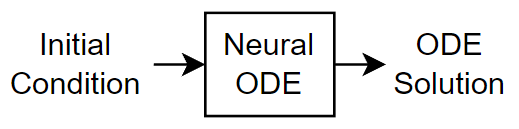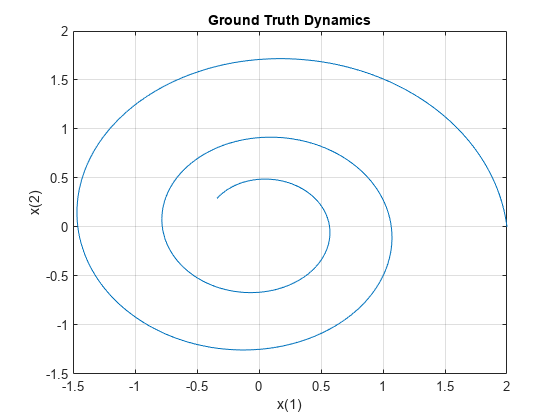# Train Neural ODE Network

This example shows how to train a neural network with neural ordinary differential equations (ODEs) to learn the dynamics of a physical system.

Neural ODEs  are deep learning operations defined by the solution of an ordinary differential equation (ODE). More specifically, neural ODE is a layer that can be used in any architecture and, given an input, defines its output as the numerical solution of the following ODE:

`${y}^{\prime }=f\left(t,y,\theta \right)$`

for the time horizon $\left({t}_{0},{t}_{1}\right)$ and with initial condition $y\left({t}_{0}\right)={y}_{0}$. The right hand size $f\left(t,y,\theta \right)$ of the ODE depends on a set of trainable parameters $\theta$, which are learnt during the training process. In this example $f\left(t,y,\theta \right)$ is modeled with a `dlnetwork` object, which is embedded into a custom layer. Typically, the initial condition ${y}_{0}$ is either the input of the entire network, as in the case of this example, or is the output of a previous layer.

This example shows how to train a neural network with neural ordinary differential equations (ODEs) to learn the dynamics $x$ of a given physical system, described by the following ODE:

${x}^{\prime }=Ax$,

where $A$is a 2 x 2 matrix.

In this example, the ODE which defines the model is solved numerically with a 4th order explicit Runge-Kutta (RK) method  in the forward pass. The backward pass uses automatic differentiation to learn the trainable parameters $\theta$, by backpropagating through each operation of the RK solver.

The example then shows how to use the learnt function $f\left(t,y,\theta \right)$ as right hand side of a model in `ode45` for computing the solution of the same model from additional initial conditions.

### Synthesize Data of the Target Dynamics

Define the target dynamics as a linear ODE model.

```x0 = [2; 0]; A = [-0.1 -1; 1 -0.1];```

Find numerical solution of the problem above. Create a set of data points to use for training using the ode45 function.

```numTimeSteps = 4000; T = 15; odeOptions = odeset('RelTol', 1.e-7, 'AbsTol', 1.e-9); t = linspace(0, T, numTimeSteps); [~, x] = ode45(@(t,y) A*y, t, x0, odeOptions); x = x';```

### Define Network

Define a `dlnetwork` object that is used as `Learnable` property in the custom layer `neuralOdeLayer`.

For each observation, `neuralOdeInternalDlnetwork` takes a vector of length `inputSize`, the size of the ODE solution, as input, it enlarges it so that it has length `hiddenSize` and then compresses it again to have length `outputSize`. The object `neuralOdeInternalDlnetwork` defines the right hand side $f\left(t,y,\theta \right)$of the ODE to be solved and the trainable parameters $\theta$ are the learnables of `neuralOdeInternalDlnetwork`. You can make the neural ode architecture more expressive, for instance by increasing the hidden size or the number of layers which define the `neuralOdeInternalDlnetwork`.

```hiddenSize = 30; inputSize = size(x,1); outputSize = inputSize; neuralOdeLayers = [ fullyConnectedLayer(hiddenSize) tanhLayer fullyConnectedLayer(hiddenSize) tanhLayer fullyConnectedLayer(outputSize) ]; neuralOdeInternalDlnetwork = dlnetwork(neuralOdeLayers,'Initialize',false); neuralOdeInternalDlnetwork.Learnables```
```ans=6×3 table Layer Parameter Value ______ _________ ____________ "fc_1" "Weights" {0×0 double} "fc_1" "Bias" {0×0 double} "fc_2" "Weights" {0×0 double} "fc_2" "Bias" {0×0 double} "fc_3" "Weights" {0×0 double} "fc_3" "Bias" {0×0 double} ```

Define the neural ODE layer which has a `dlnetwork` object as learnable parameter. The custom layer `neuralODELayer` takes a mini-batch of data which represents initial conditions and outputs the predicted dynamics by numerically solving the problem ${y}^{\prime }=f\left(t,y,\theta \right)$, where $f\left(t,y,\theta \right)$ is modeled by `neuralOdeInternalDlnetwork`.

`The value` of `neuralOdeInternalTimesteps` determines the number of timesteps that of the Runge-Kutta ODE solver internally used in the custom layer `neuralOdeLayer`. The value of `dt` is the step size employed in the internal RK method.

```neuralOdeInternalTimesteps = 40; dt = t(2); neuralOdeLayerName = 'neuralOde'; customNeuralOdeLayer = neuralOdeLayer(neuralOdeInternalDlnetwork,neuralOdeInternalTimesteps,dt,neuralOdeLayerName);```

Declare the external `dlnetwork` object and initialize the network. This initializes also the dlnetwork object in the custom `neuralOdeLayer`.

```dlnet=dlnetwork(customNeuralOdeLayer,'Initialize',false); dlnet = initialize(dlnet, dlarray(ones(inputSize,1),'CB'));```

### Specify Training Options

Specify options for the ADAM optimization.

```gradDecay = 0.9; sqGradDecay = 0.999; learnRate = 0.001;```

Train for 400 iterations with a mini-batch-size of 50.

```numIter = 1500; miniBatchSize = 200;```

Initialize the training progress plot.

```plots = "training-progress"; lossHistory = [];```

Every 50 iterations, solve with ode45 the learnt dynamics expressed as the internal dlnetwork object in the neuralOdeLayer object and display it with the ground truth in a phase diagram, this shows the training path of the learnt dynamics.

`plotFrequency = 50;`

### Train Network Using Custom Training Loop

Initialize the `averageGrad` and `averageSqGrad` parameters for ADAM solver.

```averageGrad = []; averageSqGrad= [];```

For each iteration:

• construct a mini-batch of data from the synthesized data.

• evaluate the model gradients and loss using the `dlfeval` and modelGradients functions.

• update the network parameters using the `adamupdate` function.

```if plots == "training-progress" figure(1) clf title('Training Loss'); lossline = animatedline; xlabel('Iteration') ylabel("Loss") grid on end numTrainingTimesteps = numTimeSteps; trainingTimesteps = 1:numTrainingTimesteps; start = tic; for iter=1:numIter % Create batch [dlx0, targets] = createMiniBatch(numTrainingTimesteps, neuralOdeInternalTimesteps, miniBatchSize, x); % Evaluate network and compute gradients [grads,loss] = dlfeval(@modelGradients,dlnet,dlx0,targets); % Update network [dlnet,averageGrad,averageSqGrad] = adamupdate(dlnet,grads,averageGrad,averageSqGrad,iter,... learnRate,gradDecay,sqGradDecay); % Plot loss currentLoss = extractdata(loss); if plots == "training-progress" addpoints(lossline, iter, currentLoss); drawnow end % Plot predicted vs. real dynamics if mod(iter,plotFrequency) == 0 figure(2) clf % Extract the learnt dynamics internalNeuralOdeLayer = dlnet.Layers(1); dlnetODEFcn = @(t,y) evaluateODE(internalNeuralOdeLayer, y); % Use ode45 to compute the solution [~,y] = ode45(dlnetODEFcn, [t(1) t(end)], x0, odeOptions); y = y'; plot(x(1,trainingTimesteps),x(2,trainingTimesteps),'r--') hold on plot(y(1,:),y(2,:),'b-') hold off D = duration(0,0,toc(start),'Format','hh:mm:ss'); title("Iter = " + iter + ", loss = " + num2str(currentLoss) + ", Elapsed: " + string(D)) legend('Training ground truth', 'Predicted') end end```### Evaluate Model

Use the learnt model as right hand side for the same problem with different initial conditions, solve numerically the ODE learnt dynamics with `ode45` and compare it with using the true model.

```tPred = t; x0Pred1 = sqrt([2;2]); x0Pred2 = [-1;-1.5]; x0Pred3 = [0;2]; x0Pred4 = [-2;0]; [xPred1, xTrue1, err1] = predictWithOde45(dlnet,A,tPred,x0Pred1,odeOptions); [xPred2, xTrue2, err2] = predictWithOde45(dlnet,A,tPred,x0Pred2,odeOptions); [xPred3, xTrue3, err3] = predictWithOde45(dlnet,A,tPred,x0Pred3,odeOptions); [xPred4, xTrue4, err4] = predictWithOde45(dlnet,A,tPred,x0Pred4,odeOptions);```

Plot the predicted solutions against the ground truth solutions.

```subplot(2,2,1) plotTrueAndPredictedSolutions(xTrue1, xPred1, err1, "[sqrt(2) sqrt(2)]"); subplot(2,2,2) plotTrueAndPredictedSolutions(xTrue2, xPred2, err2, "[-1 -1.5]"); subplot(2,2,3) plotTrueAndPredictedSolutions(xTrue3, xPred3, err3, "[0 2]"); subplot(2,2,4) plotTrueAndPredictedSolutions(xTrue4, xPred4, err4, "[-2 0]");```This function takes a set of initial conditions, `dlx0,` and target sequences, `targets,` and computes the loss and gradients with respect to the parameters of the network.

```function [gradients,loss] = modelGradients(dlnet, dlX0, targets) % Compute prediction of network dlX = forward(dlnet,dlX0); % Compute mean absolute error loss loss = sum(abs(dlX - targets), 'all') / numel(dlX); % Compute gradients gradients = dlgradient(loss,dlnet.Learnables); end```

This function creates a batch of observations of the target dynamics.

```function [dlX0, dlT] = createMiniBatch(numTimesteps, numTimesPerObs, miniBatchSize, X) % Create batches of trajectories s = randperm(numTimesteps - numTimesPerObs, miniBatchSize); dlX0 = dlarray(X(:, s),'CB'); dlT = zeros([size(dlX0,1) miniBatchSize numTimesPerObs]); for i = 1:miniBatchSize dlT(:, i, 1:numTimesPerObs) = X(:, s(i):(s(i) + numTimesPerObs - 1)); end end```

The function `predictWithOde45` computes the true and predicted solutions and returns them together with the corresponding error.

```function [xPred, xTrue, error] = predictWithOde45(dlnet,A,tPred,x0Pred,odeOptions) % Use ode45 to compute the solution both with the true and the learnt % models. [~, xTrue] = ode45(@(t,y) A*y, tPred, x0Pred, odeOptions); % Extract the learnt dynamics internalNeuralOdeLayer = dlnet.Layers(1); dlnetODEFcn = @(t,y) evaluateODE(internalNeuralOdeLayer, y); [~,xPred] = ode45(dlnetODEFcn, tPred, x0Pred, odeOptions); error = mean(abs(xTrue - xPred), 'all'); end function plotTrueAndPredictedSolutions(xTrue, xPred, err, x0Str) plot(xTrue(:,1),xTrue(:,2),'r--',xPred(:,1),xPred(:,2),'b-','LineWidth',1) title("x_0 = " + x0Str + ", err = " + num2str(err) ) xlabel('x1') ylabel('x2') xlim([-2 2]) ylim([-2 2]) legend('Ground truth', 'Predicted') end```

 Neural Ordinary Differential Equations, Ricky T. Q. Chen, Yulia Rubanova, Jesse Bettencourt, David Duvenaud, 2019, https://arxiv.org/abs/1806.07366

 Numerical mathematics, A Quarteroni, R Sacco, F Saleri - 2010